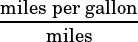College Board released a bunch of sample questions this week for the new PSAT and SAT, which will make their debuts in October 2015 and March 2016, respectively. Over the next few days, I’ll be making posts working through each question, a few at a time, and commenting on them when I feel like I have something insightful to say.

In this post, I’ll deal with questions 16 through 20 in the “calculator permitted” section. See questions 1 through 5 here, 6 through 11 here, 12 through 15 here, 21 through 26 here, and 27 through 30 here.Well, this is embarrassing for CB, but now there have been 2 questions in a row where an answer choice (and the correct answer choice, no less) is printed incorrectly. (See Question 15 in my previous post.) In this case, choices A and C, screengrabbed at right, should have || symbols, not blank squares. Someone didn’t check his or her font library carefully enough before choosing a math font!

Typo aside, this is a good question. The thing you need to know about similar triangles is that their corresponding angles are congruent. Since you have vertical angles at point C, which must be congruent to each other, you know how the corresponding angles are laid out. I’ve color coded them below.Do you see what’s going on at points B and D, and points A and E? Those are alternate interior angles of lines AB and DE, so if they’re congruent, then those lines must be parallel!

Another Heart of Algebra question, another scenario described that we need to identify an equation for. For this one, it’ll be helpful to use units. You know you want to end up with gallons, so make sure all the miles and hours units in your equation cancel out!

Hopefully it’s obvious that you need to start with “17 –” something. That right there eliminates choices C and D, amazingly.

Each remaining choice has a 50t in it. The 50, we know, is a speed, in miles per hour. The t is time, in hours. So the units of 50t is miles per hour × hours = miles. Remember that we want our final units to be gallons.

The units of 21 is miles per gallon. So between choices A and B, we’re choosing betweenand. Which of those simplifies to gallons? B does.

They’re not even asking you to solve systems of equations anymore—they’re just asking you to be able to write them. This is just the first step on medium and hard questions on the current test, but on this medium question it’s the only step. There are x cars and y trucks, so you xy should equal the total number of vehicles, 187. You also know how much each kind of vehicle has to pay in tolls. Cars pay $6.50 and trucks pay$10. So 6.5x + 10y should tell you the total amount collected in tolls, \$1338.

Your equations, then, are xy = 187, and 6.5x + 10y = 1338. That’s choice C.

OK. There a few ways we might go here. One is to just plug the (d, p) coordinates we know (9, 18.7) and (14, 20.9) into each answer choice and see which one gives us a true equation. Since calculators are allowed on this question, that might be the fastest way. You might also enter each equation into your graphing calculator and use the table function to see which equation gives you the points you want, like so:

See how Y2 has the points you want? That means the equation entered into Y2 is the right equation!

Of course, you can also use the points you’re given to find the equations algebraically. First you find the slope:…then you find the intercept using one of the points:

18.7 = 0.44(9) + b
14.74 = b

That’ll tell you that the equation you want is p = 0.44d + 14.74. If you ask me, though, using the calculator is the way to go here.

This question is essentially asking for the slope of the line of best fit, so our job is to find 2 points on the graph, and then use the slope formula: slope =.Remember that it’s fine to approximate here! The answer choices are really far apart, so even if our approximations aren’t very good, we’ll know the right answer. Using my numbers, here’s the slope calculation: